# # C++基础16-标准模板库

## # String类

### # 字符串的构造

``````
void printStr(string & str) {
cout << str << endl;
}

void BuildString() {
string str1("hello world!");
printStr(str1);
string str2(10,'x');
printStr(str2);
string str3 = str1;
printStr(str3);
string str4("hello world!", 8);
printStr(str4);
const char * ch1 = "hi,xie|this is a test";
string str5(ch1 + 3, ch1 + 11);
printStr(str5);
string str6(&str5, &str5);
printStr(str6);
string str7(str5, 3);
printStr(str7);
string str8(str5, 3,3);
printStr(str8);
string str9("hehe,notice it!", 4,7);
printStr(str9);
string str10 = str8 + str9;
printStr(str10);
}

``````
1
2
3
4
5
6
7
8
9
10
11
12
13
14
15
16
17
18
19
20
21
22
23
24
25
26
27
28
29

### # 字符串的输入

``````//get和getline对回车的区别
void GetlineAndGet() {
char info;
char info2;
cin.get(info,6);
cout << info << endl;
cin.getline(info2,6);
cout << info2 << endl;
cout << "endline" << endl;

}

//C风格字符串的输入
void InputCStyleChar() {
//C-风格字符串有3种输入方式
char info;
//读取字符串，如果遇到空格，制表符，或者回车则视为结束
cin >> info;
cout << info << endl;
//读取5个字符，在info末尾添加'\0',丢掉'\n'。
cin.getline(info,6);
cout << info << endl;
//读取5个字符，在info末尾添加'\0',将'\n'留在输入流中。
cin.get(info,6);
cout << info << endl;
cin.getline(info, 6, ':');
cout << info << endl;

}

//String字符串的输入
void InputString() {
string stuff;
cin >> stuff;
cout << stuff;
//读取字符串，如果遇到空格，制表符，或者回车则视为结束
getline(cin, stuff);
cout << stuff;
getline(cin, stuff,':');
cout << stuff;
}

//String和C风格字符串的输入
void StringAndChar() {
string sname;
char cname;
cin >> cname;
cout << cname;
cin >> sname;
cout << sname;

cout << string::npos << endl;
//注意string可以不用指定读取字符个数，但是string对象的最大允许长度是由常量string::npos指定。对于普通的交互式输入，这通常不会有问题，但是如果将整个文件读入到单个string对象中，这可能成为限制因素。
}

``````
1
2
3
4
5
6
7
8
9
10
11
12
13
14
15
16
17
18
19
20
21
22
23
24
25
26
27
28
29
30
31
32
33
34
35
36
37
38
39
40
41
42
43
44
45
46
47
48
49
50
51
52
53
54
55
1. 在C风格字符串中，getline和get对换行符的处理是不一样的，getline会读取换行符并抛弃，get会将换行符留在输入流中。

2. 使用cin会导致忽略空格符，比如前面讲到的：

``````char cname;
while (cin >> cname) {
cout << cname;
}
``````
1
2
3
4
3. String版本的getline()函数从输入中读取字符，并将其存储到目标string中，直到发生下面三种情况：

1. 到文件尾，eofbit将被设置，意味着fail()和eof()返回true。
2. 遇到分界符，从输入流中删除但不存储它。
3. 字符数达到最大允许值，将设置输入流的failbit,意味着方法fail()返回true。

### # 字符串的操作

``````    string str1("test");
string str2("this is a math test!");
cout << str2.length() << endl;
cout << str1.size() << endl;
//length()成员来自较早版本的string类，而size()则为提供STL兼容性而添加。
//默认从str2的第0个字符开始查找str1子串，并返回其首字母出现位置。
cout << str2.find(str1) << endl;
//默认从str2的第10个字符开始查找str1的字符子串，并返回其首字母出现位置。
cout << str2.find(str1,10) << endl;
//默认从str2的第10个字符开始查找str1的前n个字符子串，并返回其首字母出现位置。
//默认从str2的第10个字符开始查找字符，并返回其位置。
cout << str2.find('m',10) << endl;
//查找其子字符串或字符最后一次出现的位置
cout << str2.rfind(str1) << endl;
//查找子字符串第一次出现位置
cout << str2.find_first_of("e") << endl;
//查找子字符串最后一次出现位置
cout << str2.find_last_of("e") << endl;
//查找第一个不包含字符串中字符的位置
cout << str1.find_first_not_of("es") << endl;
//查找最后一个不包含字符串中字符的位置
cout << str1.find_last_not_of("es") << endl;
//如果没有找到，则返回string::npos
cout << (str2.find("source")!=string::npos) << endl;
``````
1
2
3
4
5
6
7
8
9
10
11
12
13
14
15
16
17
18
19
20
21
22
23
24
25

### # 字符串的种类

``````namespace pmr {
template<class _Elem,
class _Traits = char_traits<_Elem>>
using basic_string = _STD basic_string<_Elem, _Traits, polymorphic_allocator<_Elem>>;

using string = basic_string<char>;
using u16string = basic_string<char16_t>;
using u32string = basic_string<char32_t>;
using wstring = basic_string<wchar_t>;
}
``````
1
2
3
4
5
6
7
8
9
10

basic_string有四个具体化,能够使用基于类型wchar_t,char16_t,char32_t和char的字符串。

## # 智能指针模板类

### # 使用智能指针

``````#include <memory>

class ManageObj {
private:
string str;
public:
ManageObj(const string s) :str(s) {
cout << "Object is created!" << endl;
}
~ManageObj() {
cout << "Object is deleted!" << endl;
}
void What() {
cout << "str content:" << str << endl;
}
};
int main()
{
{
unique_ptr<ManageObj> uniquePtr(new ManageObj("using unique_ptr"));
uniquePtr->What();
}
{
shared_ptr<ManageObj> sharedPtr(new ManageObj("using shared_ptr"));
sharedPtr->What();
}
{
ManageObj * commonPtr1 = & ManageObj("using common ptr");
cout << "this is a temp object!" << endl;
commonPtr1->What();
}
{
ManageObj * commonPtr2 = new ManageObj("using common ptr");
commonPtr2->What();
cout << commonPtr2 << endl;
}
{
cout << "Input a address:" << endl;
ManageObj * commonPtr3 = (ManageObj *)addr;
commonPtr3->What();
cout << commonPtr3 << endl;
}
cout << "program is ended!" << endl;
system("PAUSE");
}
``````
1
2
3
4
5
6
7
8
9
10
11
12
13
14
15
16
17
18
19
20
21
22
23
24
25
26
27
28
29
30
31
32
33
34
35
36
37
38
39
40
41
42
43
44
45
46
47
48

### # 多指针赋值

1. 定义赋值运算符，执行深复制，这样两个指针指向不同对象。这种做法也是前面常用的。
2. 建立内存的所有权(ownership)概念，对于每块内存，只能有一个智能指针拥有它，赋值时转让所有权，这是auto_ptr和unique_ptr的策略，但是unique_ptr策略更严格。
3. 创建计数的指针，当有一个指针指向一块内存时，计数加1，当计数减为0时，调用delete释放内存。这是shared_ptr采用的策略。

``````unique_ptr<string> GetTempUnique_ptr(const char * s) {
unique_ptr<string> temp(new string(s));
return temp;
}
int main()
{
{
unique_ptr<string> p1(new string("hi,world!"));
unique_ptr<string> p2;
//p2 = p1;
}
{
shared_ptr<string> p1(new string("hi,world!"));
shared_ptr<string> p2;
p2 = p1;
cout << *p2 << endl;
}
{
unique_ptr<string> p3;
p3 = GetTempUnique_ptr("hello world");
cout << *p3 << endl;
}
cout << "program is ended!" << endl;
system("PAUSE");
}
``````
1
2
3
4
5
6
7
8
9
10
11
12
13
14
15
16
17
18
19
20
21
22
23
24
25

### # 注意点

1. 不存在从普通指针到智能指针的隐式转换：

``````int * idPtr1 = new int{3};
cout << *idPtr1 << endl;
shared_ptr<int> idPtr2;
//idPtr2 = idPtr1;//不能进行隐式转换
idPtr2 = shared_ptr<int>(idPtr1);
cout << *idPtr2 << endl;
//shared_ptr<int> idPtr3 = idPtr1; //不能进行隐式转换
shared_ptr<int> idPtr3(idPtr1);
cout << *idPtr3 << endl;
``````
1
2
3
4
5
6
7
8
9
2. 不要让智能指针指向非堆内存：

``````{
string str1("This is a temp string!");
shared_ptr<string> str2(&str1);
cout << *str2 << endl;
}
``````
1
2
3
4
5
3. unique_ptr比auto_ptr有优势的：

1. 把一个智能指针赋给另一个时编译器会认为非法，不会让原智能指针成为悬挂指针。
2. 把一个智能指针赋给另一个，源智能指针是个临时右值时，编译器允许这样做。
3. unique_ptr有new[]和delete[]版本，也就是可用于数组变体，auto_ptr使用delete而不是delete[]，因此只能与new一起使用。
4. 如何选择智能指针？如果要使用多个指向同一个对象的指针，应选择shared_ptr，如果编译器没有提供，可以使用Boost库提供的shared_ptr；如果不需要多个指向同一个对象的指针，则可使用unique_ptr。

## # 标准模板库

Alex Stepanov和MengLee在Hewlett-Packard实验室开发了STL，于1994年发布实现。ISO/ANSI C++委员会投票同意将其作为C++标准的组成部分。STL不是面向对象的编程，而是一种不同的编程模式——泛型编程(generic programming)。

### # 模板类vector

``````template<class _Ty,
class _Alloc = allocator<_Ty>>
class vector
: public _Vector_alloc<_Vec_base_types<_Ty, _Alloc>>
{}
``````
1
2
3
4
5

``````#include <vector>
#include <algorithm>
#include <random>
#include <chrono>

void outputArr(int pr) {
cout << pr << endl;
}

bool SortBy(int i1, int i2) {
if (i1 > i2) {
return true;
}
return false;
}

int main()
{
cout << "Input number of vector array:" << endl;
int n;
cin >> n;
vector<int> intArr(n);
for (int intat = 0; intat < n; intat++) {
intArr[intat] = intat;
cout << intArr[intat] << endl;
}
cout << "vector size:" << intArr.size() << endl;

vector<int> intArr2(n);
for (int intat = 0; intat < n; intat++) {
intArr2[intat] = intat+100;
cout << intArr2[intat] << endl;
}
intArr.swap(intArr2);
cout << "-------------vector swap:---------------" << endl;

vector<int>::iterator iter;
for (iter = intArr.begin(); iter != intArr.end(); iter++) {
cout << *iter << endl;
}

intArr.push_back(100);
cout << "vector size:" << intArr.size() << endl;

intArr.erase(intArr.begin() + 1, intArr.begin() + 3);//不包括intArr.begin()+3元素
intArr.insert(intArr.begin() + 2, intArr.begin() + 1, intArr.end());

for (auto pr = intArr.begin(); pr != intArr.end(); pr++) {
cout << (*pr) << endl;
}

cout << "------------------random shuffle:--------------" << endl;
//random_shuffle(intArr.begin(),intArr.end()); //该函数在C++14中deprecated，在C++17中removed
unsigned seed = chrono::system_clock::now().time_since_epoch().count();
shuffle(intArr.begin(), intArr.end(),default_random_engine(seed));

for_each(intArr.begin(),intArr.end(),outputArr);

cout << "------------------sort numbers:--------------" << endl;

sort(intArr.begin(),intArr.end());

for (auto x : intArr) {
outputArr(x);
}
//想自己定义排序规则重载int的<操作符。

cout << "------------------sort by self define:--------------" << endl;
sort(intArr.begin(),intArr.end(),SortBy);
for_each(intArr.begin(),intArr.end(),outputArr);

cout << "program is ended!" << endl;
system("PAUSE");
}
``````
1
2
3
4
5
6
7
8
9
10
11
12
13
14
15
16
17
18
19
20
21
22
23
24
25
26
27
28
29
30
31
32
33
34
35
36
37
38
39
40
41
42
43
44
45
46
47
48
49
50
51
52
53
54
55
56
57
58
59
60
61
62
63
64
65
66
67
68
69
70
71
72
73
74
75
76
77

vector有size()返回容器中的元素的数目，swap()交换两个容器的内容，begin()返回一个指向容器中第一个元素的迭代器，end()返回一个表示超过容器尾的迭代器。注意迭代器可以是一个广义指针，也可以是一个可对其指向类似指针的操作，比如有解除引用(operator*())和递增(operator++())的对象。通过将指针广义化为迭代器，让STL能够为各种不同的容器类(包括那些简单指针无法处理的类)提供统一的接口。每个容器类都定义了一个合适的迭代器，该迭代器的类型是一个名为iterator的typedef，其作用域为整个类。

### # 基于范围的for循环

``````    int arr = {
1,2,3,4,5
};
for (auto & x : arr) {
cout << x << endl;
x += 10;
}
for (auto x : arr) {
cout << x << endl;
}
``````
1
2
3
4
5
6
7
8
9
10

## # 泛型编程

STL是面向泛型编程，面向对象编程关注的是编程的数据方面，而泛型编程关注的是算法。它们之间的共同点是抽象和创建可重用代码，但理念绝然不同。泛型编程旨在编写独立于数据类型的代码，在C++中，完成通用程序的工具是模板，模板使得能按泛型定义函数或类，而STL在模板基础上使通用算法更进了一步。

### # 何为迭代器

``````struct Node {
int value;
Node * p_next;
};

int * findValueA(int * ptr,int n,const int & val) {
for (int i = 0; i < n; i++) {
if (ptr[i] == val) {
return &ptr[i];
}
}
return 0;
}

Node * findValueB(Node * head,const int & val ) {
//	if (head->value == val) {
//	}
//}
for (Node * ptr = head; ptr != 0; ptr = ptr->p_next) {
if (ptr->value == val) {
return ptr;
}
}
return 0;
}
``````
1
2
3
4
5
6
7
8
9
10
11
12
13
14
15
16
17
18
19
20
21
22
23
24
25
26
27
28

``````typedef int * iteratorA;
iteratorA findValueA(iteratorA begin,iteratorA end,const int & val) {
for (iteratorA ar = begin; ar != end;ar++) {
if (*ar == val) {
return ar;
}
}
return 0;
}

class iteratorB {
Node * pt;
public:
iteratorB():pt(0) {}
iteratorB(Node * pn):pt(pn) {}
int operator*() {
return pt->value;
}
iteratorB& operator++() {//for ++it
pt = pt->p_next;
return *this;
}
iteratorB operator++(int) { //for it++
iteratorB tmp = *this;
pt = pt->p_next;
return tmp;
}
bool operator==(iteratorB b1) {
if (pt->value == *b1) {
return true;
}
return false;
}
bool operator!=(iteratorB b1) {
return !operator==(b1);
}
};

iteratorB findValueB(iteratorB head,const int & val ) {
for (iteratorB ptr = head; ptr != 0; ptr++) {
if (*ptr == val) {
return ptr;
}
}
return 0;
}

``````
1
2
3
4
5
6
7
8
9
10
11
12
13
14
15
16
17
18
19
20
21
22
23
24
25
26
27
28
29
30
31
32
33
34
35
36
37
38
39
40
41
42
43
44
45
46
47
48

1. 每个容器类(vector,list,deque等)定义了相关的迭代器类型，对于其中的某个类型，迭代器可能是指针，对于另一个类，则可能是对象。不管实现方式如何，迭代器都提供所需操作比如*和++。
2. 每个容器类应该有一个超尾标记，当迭代器递增到超越容器的最后一个值后，这个值被赋给迭代器。
3. 每个容器类应该有begin()和end()方法，它们分别返回一个指向容器的第一个元素和超尾位置的迭代器。
4. 每个容器类都使用++操作，让迭代器从指向第一个元素逐步指向超尾位置，从而遍历容器中的每一个元素。

### # 迭代器类型

STL定义了5中迭代器：输入迭代器，输出迭代器，正向迭代器，双向迭代器和随机访问迭代器。这5中迭代器都可以执行解除引用(*)，也可进行比较(==或!=)。

### # 迭代器层次结构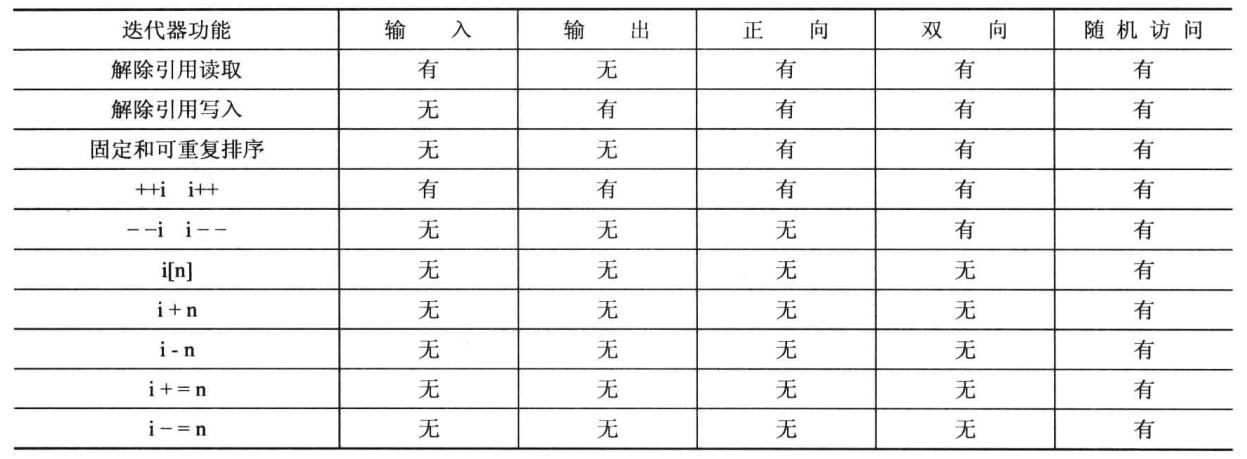### # 概念，改进和模型

STL有若干个用C++语言无法表达的特性，比如迭代器的种类，虽然可以设计具有正向迭代器特征的类，但不能让编译器将算法限制为只使用这个类，因为正向迭代器是一系列要求而不是类型。STL使用概念(concept)来描述这种一系列的要求；概念可以有类似继承的关系，比如双向迭代器确实继承了正向迭代器的功能，但是却不能称之为继承，使用改进(refinement)来表示这种概念上的继承。因此双向迭代器是正向迭代器概念的一种改进；概念的具体实现被称为模型(model)。

#### # 指针作为迭代器

``````#include <ctime>
#include <vector>
#include <algorithm>
#include <iterator>
#include <queue>

#define printArr(arr) for (int one : arr) {cout << one << endl;}

int main()
{
srand(time(0));
const int Length = 20;
int arr[Length];
vector<int> v1(Length);
vector<int> v2(5);
deque<int> v3;
ostream_iterator<int, char> out_iter(cout, "\n");
for (int i = 0; i < Length; i++) {
arr[i] = rand() % 100;
v1[i] = rand() % 100;
}

cout << "-------------------array origin:----------------" << endl;
printArr(arr);
cout << "-------------------vector origin:----------------" << endl;
printArr(v1);
cout << "-------------------sort array：----------------" << endl;
sort(arr, arr + Length);
printArr(arr);
cout << "-------------------copy to vector----------------" << endl;
//这些值将覆盖v1的值，而且假设v1有足够的空间，copy()无法自动根据源容器值大小调整目标容器的长度。
copy(arr, arr + Length, v1.begin());
printArr(v1);
cout << "-------------------back_insert arr into v2:----------------" << endl;
copy(arr, arr + Length, back_insert_iterator<vector<int>>(v2));
printArr(v2);
cout << "-------------------front_insert arr into deque v3:----------------" << endl;
copy(arr, arr + Length, front_insert_iterator<deque<int>>(v3));
while (!v3.empty()) {
cout << v3.front() << endl;
v3.pop_front();
}
cout << "-------------------insert_iterator arr into deque v2:----------------" << endl;
copy(arr,arr+Length,insert_iterator<vector<int>>(v2,v2.begin()));
printArr(v2);
cout << "-------------------use ostream_iterator output:----------------" << endl;
*out_iter++ = 15;
//like cout << 15 <<"\n";
cout << "-------------------display arr----------------" << endl;
copy(arr, arr + Length,out_iter);
cout << "-------------------display vector----------------" << endl;
copy(v1.begin(), v1.end(),out_iter);
cout << "-------------------display reverse vector----------------" << endl;
copy(v1.rbegin(), v1.rend(),out_iter);
//rbeign()和end()返回一样的指针，但是类型不同，rbegin是reverse_iterator，end是iterator。同理，rend()和begin()也返回相同的值，但是类型不同。
//需要对反向指针做一种特殊补偿，假设rp是一个被初始化为v1.rbegin()指针，那么rp是指向超尾，无法进行*rp操作，同样如果rend()是第一个元素的位置，则copy必须提早一个位置停止，因为区间的结尾处不包括区间中。
//反向指针通过先递减再解决引用解决了这两个问题，加入rp指向位置6，那么*rp将是位置5的值。
cout << "-------------------display vector----------------" << endl;
copy(v1.begin(), v1.end(),ostream_iterator<int,char>(cout," "));
cout << endl;
cout << "-------------------input vector v2:----------------" << endl;
copy(istream_iterator<int, char>(cin), istream_iterator<int, char>(), v2.begin());
//第一个参数，定义一个连接到标准输入的istream_iterator。
//第二个参数，定义istream_iterator时不指定istream对象代表end-of-file。实际使用ctrl z
printArr(v2);
}

``````
1
2
3
4
5
6
7
8
9
10
11
12
13
14
15
16
17
18
19
20
21
22
23
24
25
26
27
28
29
30
31
32
33
34
35
36
37
38
39
40
41
42
43
44
45
46
47
48
49
50
51
52
53
54
55
56
57
58
59
60
61
62
63
64
65
66
67

copy()将数据从一个容器复制到另一个容器中。它以迭代器方式实现，从实例中看到，它不仅可以在普通数组和矢量之间操作，还能应用到ostream_iterator来将数据显示到标准输出，应用到istream_iterator来从标准输入获取数据。

copy()不能自动根据源容器来调整目标容器的长度，有三种插入迭代器解决了这个问题:

1. back_insert_iterator将元素插入到容器尾部
2. front_insert_iterator将元素插入到容器前端，只有deque类和list类提供的push_front成员函数支持。
3. insert_iterator将元素插入到指定位置的前面。

### # 容器种类

STL具有容器概念和容器类型。概念是具有名称(如容器，序列容器，关联容器等)的通用类别，类型是可用于创建具体容器对象的模板。以前的11个容器类型是deque,list,queue,priority_queue,stack,vector,map,multimap,set,multiset,bitset。C++11新增了forward_list,unordered_map,unordered_multimap,unordered_set和unordered_multiset，暂不将bitset视为容器，而视为一种独立的类别。

#### # 容器概念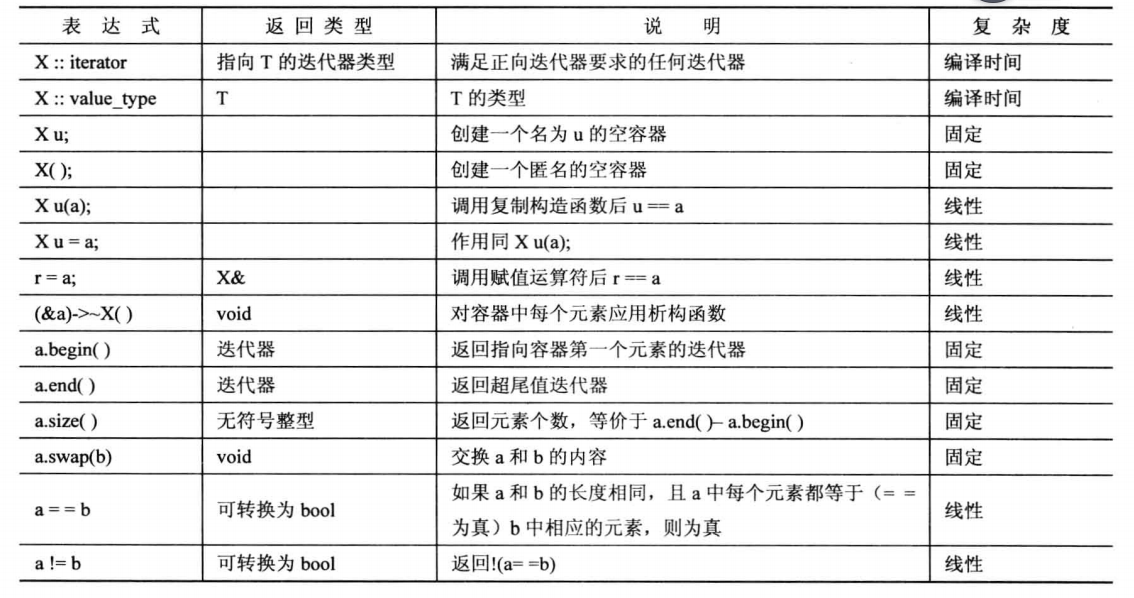#### # C++11新增的容器要求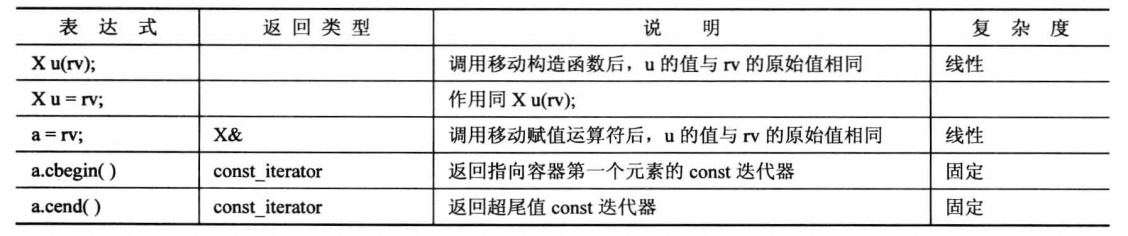#### # 序列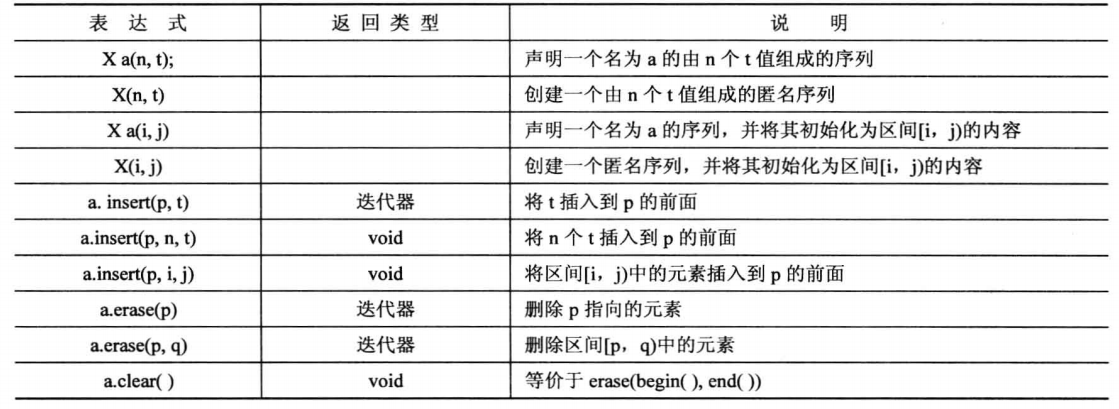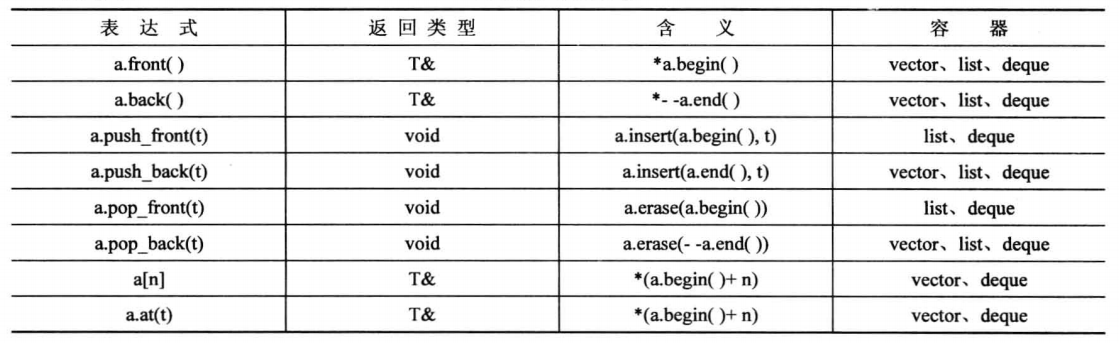#### # 具体序列类型

##### # vector

vector是数组的一种类表示，它提供了自动内存管理，可以动态的改变vector对象的长度，随着元素的添加和删除而增加和缩小。它提供了对元素的随机访问，在尾部添加和删除元素的时间是固定的，在头部或中间插入和删除元素的复杂度为线性时间。vector还可反转容器，提供rbegin()和rend()，它们返回的容器类型是reverse_iterator。vector模板是最简单的序列类型，除非有特殊要求使用其他类型，否则默认使用这种类型。

##### # deque

deque模板类表示双端队列(double-ended queue)，通常被简称为deque。它支持随机访问，同vector的区别是它从对象的开始位置插入和删除的时间是固定的，如果多数操作发生在序列的起始和结尾处应该考虑使用deque结构。

##### # list

list模板类表示双向链表，除了第一个和最后一个元素外，每个元素都与前后的元素相链接，意味着可以双向遍历链表。list和vector的关键区别在于，list在链表中任一位置进行插入和删除的时间都是固定的,vector仅在结尾是插入和删除的时间是固定的。vector强调的是随机快速访问而list强调元素的快速插入和删除。list也可以反转容器，但是不支持数组表示法和随机访问。下图为list的成员函数。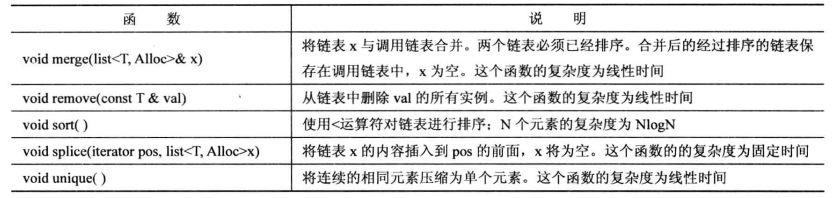``````#include <list>

#define printArr(arr) for (int one : arr) {cout << one << endl;}

int main()
{
list<int> intList(5, 10);
int intArr = {
2,4,6,8,10
};
list<int> intList2;
list<int> intList3(3,6);
intList3.insert(intList3.end(), 20);
cout << "---------insert intArr to intList!---------" << endl;
intList2.insert(intList2.begin(),intArr,intArr+5);
printArr(intList2);
cout << "---------insert intList to intList2 begin!---------" << endl;
intList2.insert(intList2.begin(),intList.begin(),intList.end());
printArr(intList2);
cout << "---------splice intList to intList2 end!---------" << endl;
intList2.splice(intList2.end(), intList);
printArr(intList2);
cout << "---------after splice,intList content:----------" << endl;
printArr(intList);
cout << "---------unique intlist2:------------" << endl;
intList2.unique();
printArr(intList2);
cout << "---------intList2 sort:-----------" << endl;
intList2.sort();
printArr(intList2);
cout << "----------merge intList3 into intList2:-----------" << endl;
intList2.merge(intList3);
//两个链表必须已经排序。
printArr(intList2);
cout << "---------intList3 content:-----------" << endl;
printArr(intList3);
}
``````
1
2
3
4
5
6
7
8
9
10
11
12
13
14
15
16
17
18
19
20
21
22
23
24
25
26
27
28
29
30
31
32
33
34
35
36
37
38
##### # forward_list

C++11新增类，实现了单链表。在该链表中，每个节点只链接到下一个节点，而没有链接到前一个节点。所以它只需要正向迭代器，而不需要双向迭代器。不同于vector和list，forward_list是不可反转的容器。

##### # queue

queue模板类是一个适配器类，类似ostream_iterator模板让输出流使用迭代器接口，queue模板则是队列接口。queue模板的限制比deque更多，它不仅不允许随机访问队列元素，也不允许遍历队列。它仅支持如下操作：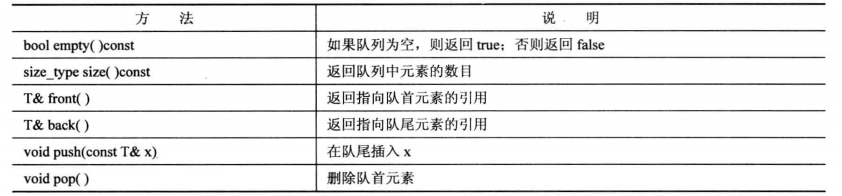##### # priority_queue

``````priority_queue<int> pq1;
priority_queue<int> pq2(greater<int>);
//greater<>是一个预定义的函数对象。
``````
1
2
3
##### # stack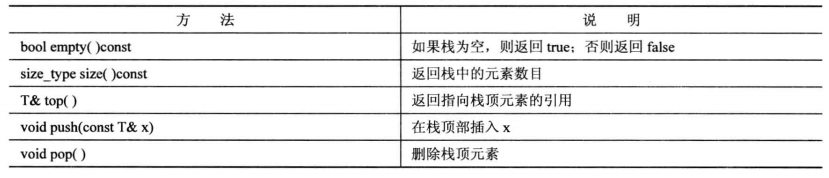### # 关联容器

STL提供了4种关联容器：set,multiset,map和multimap。前两种是在头文件set定义，后两种是在map中定义。

#### # set

``````#include <set>
#include <iterator>
#include <algorithm>

#define printArr(arr) for (int one : arr) {cout << one << endl;}

int main()
{
int intArr = {10,6,14,7,5};
set<int> s1 = {5,3,9,7,9};
//第二个模板参数是可选的，可用于指示用来对键进行排序的比较函数或对象。默认将是用less<>
cout << "----------s1 content:---------" << endl;
printArr(s1);
set<int, greater<int>> s2 = {8,4,6,10,5};
cout << "----------s2 content:---------" << endl;
printArr(s2);
cout << "----------s3 content:---------" << endl;
set<int> s3(intArr,intArr+5);
printArr(s3);
cout << "----------s4 content:---------" << endl;
set<int,greater<int>> s4(intArr,intArr+5);
printArr(s4);

//得到s1和s2的并集
cout << "----------s5 is s1 and s3's union oper,s5 content:---------" << endl;
//set_union，set_intersection，set_difference需要两个set容器要按同一个顺序排列。
set<int> s5;
set_union(s1.begin(),s1.end(),s3.begin(),s3.end(),insert_iterator<set<int>>(s5,s5.begin()));
printArr(s5);
cout << "----------output s1 and s3's union oper:----------" << endl;
ostream_iterator<int, char> out(cout, "\n");
set_union(s1.begin(),s1.end(),s3.begin(),s3.end(),out);
cout << "----------output s1 and s3's intersection oper:----------" << endl;
set_intersection(s1.begin(),s1.end(),s3.begin(),s3.end(),out);
cout << "----------output s1 and s3's difference oper:----------" << endl;
set_difference(s1.begin(),s1.end(),s3.begin(),s3.end(),out);
cout << "----------Get s1 container's range walue" << endl;
copy(s1.upper_bound(5), s1.lower_bound(8), out);
}

``````
1
2
3
4
5
6
7
8
9
10
11
12
13
14
15
16
17
18
19
20
21
22
23
24
25
26
27
28
29
30
31
32
33
34
35
36
37
38
39
40

#### # map

``````#include <map>
#include <iterator>
#include <algorithm>

#define printArr(arr) for (auto one : arr) {cout << one << endl;}

int main()
{
typedef int KeyType;
typedef multimap<KeyType,string> CodeMap;
typedef pair<KeyType, string> Pair;
//第三个参数同set一样指定对键进行排序的比较函数和对象
CodeMap cityCode;
cityCode.insert(Pair(1,"Beijing"));
cityCode.insert(Pair(2,"ShangHai"));
cityCode.insert(Pair(2,"ShangHai2"));
cityCode.insert(Pair(2,"ShangHai3"));
cityCode.insert(Pair(3,"GuangZhou"));
cityCode.insert(Pair(4,"ShenZhen"));
cityCode.insert(Pair(5,"ChengDu"));
cityCode.insert(Pair(6,"HangZhou"));

cout << cityCode.count(2) << endl;
for (auto one : cityCode) {
cout << "code:" << one.first << "  city name:" << one.second <<endl;
}
for (CodeMap::iterator it = cityCode.begin(); it != cityCode.end(); it++) {
cout << "code:" << (*it).first << "  city name:" << (*it).second <<endl;
}
cout << "-----------value with key is 2:-------------" << endl;
auto result = cityCode.equal_range(2);
CodeMap::iterator it;
for (it = result.first; it != result.second; it++) {
cout << (*it).second << endl;
}
}

``````
1
2
3
4
5
6
7
8
9
10
11
12
13
14
15
16
17
18
19
20
21
22
23
24
25
26
27
28
29
30
31
32
33
34
35
36
37

## # 函数对象

``````class Multiply {
private:
double factor;
public:
Multiply(double _factor) :factor(_factor) {
}
double operator()(double x) {
return x*factor;
}
};

double Multiply2(double x) {
return 10 * x;
}

template<typename F>
void ShowResult(F f) {
cout << f(2) << endl;
}

int main()
{
Multiply m1(5);
ShowResult(m1);
ShowResult(Multiply2);
}

``````
1
2
3
4
5
6
7
8
9
10
11
12
13
14
15
16
17
18
19
20
21
22
23
24
25
26
27

### # 函数符细化概念

1. 生成器(generator)是不用参数就可以调用的函数符。
2. 一元函数(unary function)是用一个参数可以调用的函数符。
3. 二元函数(binary function)是用两个参数可以调用的函数符。
4. 返回bool值得一元函数是谓词(predicate)。
5. 返回bool值得二元函数是二元谓词(binary predicate)。
``````#include <list>

#define printArr(arr) for (auto one : arr) {cout << one << endl;}

bool filter(int n) {
if (n > 60) {
return true;
}
return false;
}

template<typename T>
class filter2 {
private:
T cutvalue;
public:
filter2(T _cutvalue) :cutvalue(_cutvalue){}
bool operator()(T x) {
if (x > cutvalue) {
return true;
}
return false;
}
};

int main()
{
int vals = { 50,60,30,43,76,23,54,98,87,65 };
list<int> list1(vals, vals + 10);
list<int> list2(vals, vals + 10);
list1.remove_if(filter);
printArr(list1);
cout << "----------list2 value:--------" << endl;
filter2 filter2Instance(70);
list2.remove_if(filter2Instance);
printArr(list2);
}

``````
1
2
3
4
5
6
7
8
9
10
11
12
13
14
15
16
17
18
19
20
21
22
23
24
25
26
27
28
29
30
31
32
33
34
35
36
37
38

list模板中的remove_if成员函数需要一个谓词作为参数，该函数将谓词应用在该容器内的每个元素，如果使用普通函数的话，因为谓词只能有一个参数，则只能将标准值写死在该函数中，但是如果使用函数符，则在对象初始化阶段指定该标准值，这样类函数符更像是一个函数适配器，使函数能够满足不同的接口。

### # 预定义的函数符

STL定义了多个基本函数符，它们将执行类似相加，比较是否相等操作。提供这些函数对象是为了支持将函数作为参数的STL函数。

``````#include <vector>
#include <functional>

#define printArr(arr) for (auto one : arr) {cout << one << endl;}

int op_increase (int i) { return ++i; }

int main()
{
int vals = { 50,60,30,43,76,23,54,98,87,65 };
vector<int> v1(vals,vals+10);
vector<int> v2(vals,vals+10);
ostream_iterator<int, char> out(cout, " ");
cout << "--------increase v1 and insert into v2---------" << endl;
transform(v1.begin(), v1.end(), v2.begin(), op_increase);
cout << "--------v1 value--------" << endl;
printArr(v1);
cout << "--------v2 value--------" << endl;
printArr(v2);
cout << "--------v1 and v2 minus---------" << endl;
transform(v1.begin(), v1.end(), v2.begin(), out , minus<int>());
}

``````
1
2
3
4
5
6
7
8
9
10
11
12
13
14
15
16
17
18
19
20
21
22
23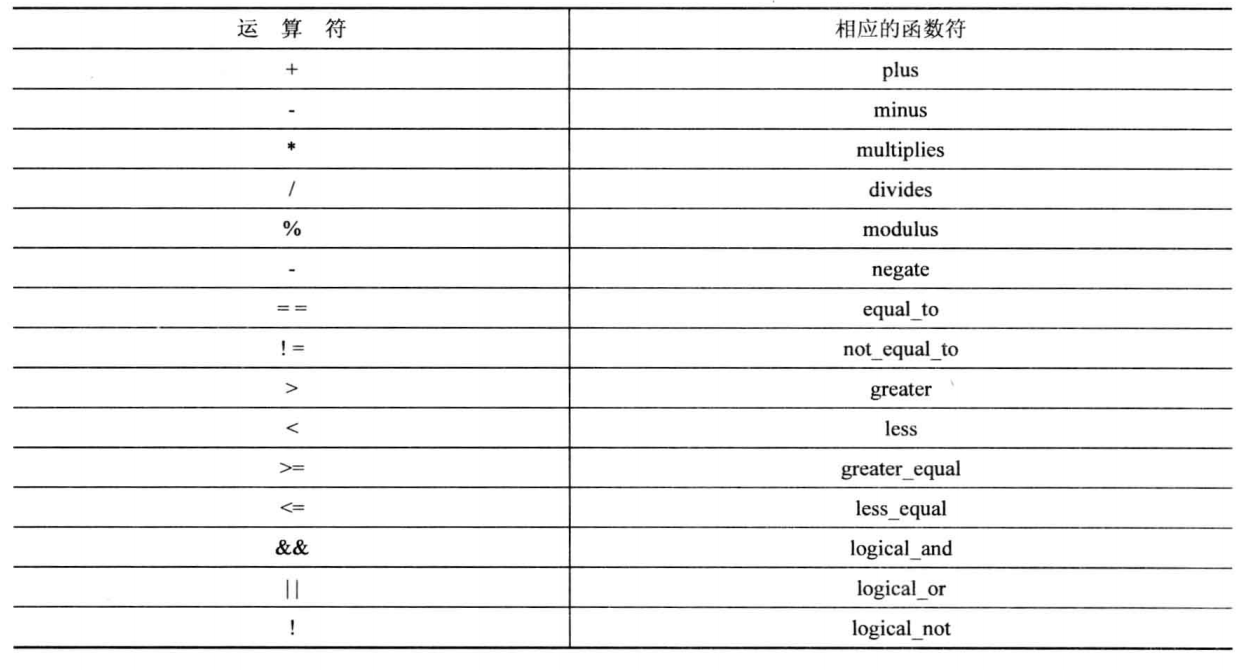### # 函数适配器

``````int main()
{
int vals = { 50,60,30,43,76,23,54,98,87,65 };
vector<int> v1(vals,vals+10);
ostream_iterator<int, char> out(cout, " ");
cout << "--------function adaptor ---------" << endl;
//transform(v1.begin(), v1.end(),out, bind1st(divides<int>(),100));
transform(v1.begin(), v1.end(), out, bind(divides<int>(), 100, placeholders::_1));
//transform(v1.begin(), v1.end(),out, bind2nd(divides<int>(),100));
transform(v1.begin(), v1.end(), out, bind(divides<int>(),placeholders::_1, 100));
}

``````
1
2
3
4
5
6
7
8
9
10
11
12

## # 算法

STL包含很多处理容器的非成员函数，前面介绍过一些:sort(),copy(),find(),random_shuffle(),set_union(),set_intersection(),set_difference()和transform()。它们的总体设计是相同的，都使用迭代器来标识要处理的数据区间和结果的放置位置。有些函数还接受一个函数对象参数，并使用它来处理数据。

### # 算法组

STL将算法库分成四组：

1. 非修改式序列操作
非修改式序列操作对区间中的每个元素进行操作，这些操作不修改容器的内容，比如find()和for_each()属于这类。
2. 修改式序列操作
修改式序列操作也对区间中的每个元素进行操作。然而它们可以修改容器中的内容，可以修改值，也可以修改值得排列顺序。transform(),random_shuffle()和copy()属于这类。
3. 排序和相关操作
排序和相关操作包括多个排序函数(包括sort())和其他各种函数，包括集合操作。
4. 通用数字运算
数字操作包括将区间的内容累积，计算两个容器的内部乘积等等，通常这些都是数组的操作特性。

### # 算法的通用特征

STL函数使用迭代器和迭代区间。从函数原型可知有关参数的假设，比如copy的函数定义如下：

``````
template<class InputIterator, class OutputIterator>
OutputIterator copy (InputIterator first, InputIterator last, OutputIterator result)
{
while (first!=last) {
*result = *first;
++result; ++first;
}
return result;
}

``````
1
2
3
4
5
6
7
8
9
10
11

1. 就地算法(in-place algorithm)。比如sort(),函数完成时结果被放在原始数据的位置上。
2. 复制算法(copying algorithm)。比如copy(),将结果发送到另一个位置上。

### # STL和string类

string类虽然不是STL的组成部分，但设计它时考虑到了STL。比如它包含begin(),end(),rbegin(),rend()等成员，因此可以使用STL接口。来看下面的实例：

``````int main()
{
string str1;
cout << "Enter a string :" << endl;
while (cin >> str1 && str1 != "quit") {
cout << "Permutations of" << str1 << endl;
sort(str1.begin(), str1.end());
do {
cout << str1 << endl;
} while (next_permutation(str1.begin(), str1.end()));
cout << "Enter next string:" << endl;
}
cout << "quit!" << endl;
return 0;
}
``````
1
2
3
4
5
6
7
8
9
10
11
12
13
14
15

### # 函数和容器方法

``````#include <algorithm>
#include <list>

#define printArr(arr) for (auto one : arr) {cout << one << endl;}

int main()
{
int ar = { 50,60,30,43,76,23,54,98,30,65 };
list<int> la(ar, ar + 10);
list<int> lb(la);
la.remove(30);
cout << "la size:" << la.size() << endl;
auto last = remove(lb.begin(), lb.end(),30);
cout << "lb size:" << lb.size() << "  lb content:" << endl;
printArr(lb);
cout << "---------erase end element--------" << endl;
lb.erase(last, lb.end());
printArr(lb);
}

``````
1
2
3
4
5
6
7
8
9
10
11
12
13
14
15
16
17
18
19
20

### # 使用STL

STL作为一个库，其组成部分被设计成协同工作。STL组件也是创建其他工具的基本部件。使用STL时要尽可能减少要编写的代码，STL通用，灵活的设计将节省大量工作。STL设计者本身就是关心效率的算法人员，算法是经过仔细选择的，并且是内联的。下面通过一个实例来做展示下STL的威力。假设要写一个程序，让用户输入单词，希望最后得到一个按输入顺序排列的单词列表，一个按字母顺序排列的单词列表（忽略大小写），并记录每个单词背输入的次数。

``````#define printArr(arr) for (auto one : arr) {cout << one << endl;}

char toLower(char ch) {
}

string & ToLower(string & st) {
transform(st.begin(), st.end(), st.begin(), toLower);
return st;
}

int main()
{
vector<string> words;
string input;
while (cin >> input && input != "quit") {
words.push_back(input);
}

//按字母顺序排列并忽略大小写
set<string> wordset;
transform(words.begin(), words.end(), insert_iterator<set<string>>(wordset, wordset.begin()), ToLower);

map<string, int> wordmap;
for (auto one : wordset) {
cout << one << endl;
wordmap[one] = count(words.begin(), words.end(), one);
}
for (auto one : wordmap) {
cout << "word:" << one.first << "  count:" << one.second << endl;
}
}

``````
1
2
3
4
5
6
7
8
9
10
11
12
13
14
15
16
17
18
19
20
21
22
23
24
25
26
27
28
29
30
31
32
33

## # 其他库

C++还提供其他一些类库，更加专用，比如complex为复数提供了类模板complex，包含用于float,long和long double的具体化，这个类提供了标准的复数运算及能够处理复数的标准函数。C++11新增的头文件random提供了更多的随机数功能。还有前面介绍的valarray提供了模板类valarray，这个类被设计成用于表示数值数组，支持各种数值数组操作。

### # vector,valarray和array

C++为何提供三个数组模板：vector，valarray和array。这些类由不同的小组开发，用于不同的目的。vector模板类是一个容器类和算法系统的一部分，它支持面向容器的操作，如排序，插入，重新排列，搜索，将数据转移到其他容器中。而valarray类模板时面向数值计算的，不是STL一部分，它没有push_back()和insert()方法，但为很多数学运算提供了一个简单，直观的接口。最后，array是为替代内置数组而设计的，它通过提供更好，更安全的接口，让数组更紧凑，效率更高，它表示长度固定的数组，因此也不支持push_back()和insert()，但提供了多个STL方法，包括begin()，end()，rbegin()，rend()，使得很容易将STL算法用于array对象。

``````#define printArr(arr) for (auto one : arr) {cout << one << endl;}

int main()
{
int arrInit{ 1,2,3,4,5 };
vector<int> vec1(arrInit,arrInit+5);
array<int, 5> arr1{6,7,8,9,10};
valarray<int> valarr1{12,11,14,13,15};
valarray<int> valarr2{21,22,23,24,25};
valarray<int> valarr3;
transform(vec1.begin(), vec1.end(), arr1.begin(),vec1.begin(),plus<int>());
transform(arr1.begin(), arr1.end(), vec1.begin(),arr1.begin(),plus<int>());
valarr3 = valarr1 + valarr2;
valarr3 = valarr1 * valarr2;
transform(vec1.begin(), vec1.end(), vec1.begin(), bind(multiplies<int>(), placeholders::_1, 10));
valarr3 = 10 * valarr1;
valarr3 = log(valarr1);
//	valarr3 = valarr1.apply(log);
//valarr3 = 10*(valarr1+valarr2)/2+valarr1*cos(valarr2);

//	cout << valarr1.max() << endl;
printArr(valarr1);
cout << "--------sort this array------" << endl;
//sort(&valarr1,&valarr1);
//上面这种做法可能会出问题，建议使用如下代码
sort(begin(valarr1),end(valarr1));
printArr(valarr1);

cout << "--------valarray 4 content:----------" << endl;
valarray<bool> valarr4 = valarr1 > 13;
printArr(valarr4)

cout << "--------use slice to point int number---------" << endl;
valarr1[slice(1, 5, 2)] = 100;
printArr(valarr1);
}

``````
1
2
3
4
5
6
7
8
9
10
11
12
13
14
15
16
17
18
19
20
21
22
23
24
25
26
27
28
29
30
31
32
33
34
35
36
37

``````const int SIZE = 12;
const int COLS = 3;
const int ROWS = 4;
typedef valarray<int> vint;

void show(const vint & v, int cols) {
for (int i = 0; i < v.size(); i++) {
cout.setf(ios::left);
cout.width(5);
cout << v[i];
if (i%cols == cols - 1) {
cout << endl;
}
else {
cout << " ";
}
}
}
auto GetColumn(int col = 0) {
if (COLS < col) {
cout << "beyond the Max columns" << endl;
return slice();
}
return slice(col - 1, ROWS, COLS);
}
auto GetRow(int row) {
if (ROWS < row) {
cout << "beyond the Max rows" << endl;
return slice();
}
return slice((row-1)*COLS, COLS, 1);
}

void showColumn(const vint & v, int col) {
show(vint(v[GetColumn(col)]),1);
}
void showRow(const vint & v, int row) {
show(vint(v[GetRow(row)]),COLS);
}

int main()
{
vint valarr(SIZE);
for (int i = 0; i < SIZE; i++) {
valarr[i] = rand() % 10;
}
vint valarr2(SIZE);
for (int i = 0; i < SIZE; i++) {
valarr2[i] = rand() % 10;
}

//valarr[GetColumn(2)] = 0;
cout << "------------show valarr--------------" << endl;
show(valarr, COLS);
cout << "------------show valarr2--------------" << endl;
show(valarr2, COLS);
valarr[GetRow(3)] += valarr2[GetRow(3)];
cout << "------------show valarr--------------" << endl;
show(valarr, COLS);
cout << "------------show column--------------" << endl;
//valarr[GetRow(2)] = 1;
showColumn(valarr, 3);
showRow(valarr, 4);
}

``````
1
2
3
4
5
6
7
8
9
10
11
12
13
14
15
16
17
18
19
20
21
22
23
24
25
26
27
28
29
30
31
32
33
34
35
36
37
38
39
40
41
42
43
44
45
46
47
48
49
50
51
52
53
54
55
56
57
58
59
60
61
62
63
64
65
66
67

### # 模板initializer_list

``````class Number {
double doubleValue;
int IntValue;
public:
Number(){};
Number(initializer_list<int> li) {
cout << "this is for initializer_list"<< endl;
for (auto one : li) {
cout << one << endl;
}
};
Number(double dv,int iv): doubleValue(dv),IntValue(iv){};
~Number() {};
void GetValue() {
cout << doubleValue << " " << IntValue << endl;
}

};

int main()
{
Number n1{10,2};
n1.GetValue();

}

``````
1
2
3
4
5
6
7
8
9
10
11
12
13
14
15
16
17
18
19
20
21
22
23
24
25
26

## # STL总结

STL是一个容器类模板，迭代器类模板，函数对象模板和算法函数模板的集合，它们的设计是一致的，都是基于泛型编程原则的。算法通过使用模板，从而独立于所存储的对象的类型；通过使用迭代器接口，从而独立于容器的类型。迭代器是广义指针。

STL使用术语"概念"来描述一组要求。例如，正向迭代器的概念包含这样的要求，即正向迭代器能够被解除引用，以便读写，同时能够被递增。概念真正的实现方式被称为概念的"模型"。例如，正向迭代器概念可以是常规指针或导航链表的对象。基于其他概念的概念叫做"改进"。双向迭代器是正向迭代器的改进。